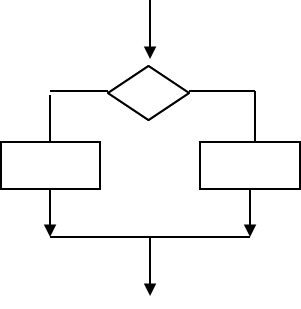# 6 Coding Puzzles Teach You How to Read IF statements

[vc_row][vc_column width=”1/1″][vc_single_image image=”9081″ border_color=”grey” img_link_large=”” img_link_target=”_self” img_size=”150×225″ alignment=”center”][vc_column_text]This blog continues our series of C# coding brainteasers, which teach you how to read code without compiling it. This blog will provide 6 puzzles on IF statements. The puzzles will help you learn practical programming skills.This is how an IF statement works.  An IF statement runs code based on a test. This test results in true or false. When it results in true on or more statements run. Optionally alternative statements can run if the test results in false. IF statements are also called conditional statements, since code is executed based on value of the condition (test). IF statements are frequently used in all levels of programming.

Try to solve these 6 IF statements with your C# or other coding knowledge. Just predict the output without executing the code. If you need some help, check our C# course.  Write your answer in the comments below. Or add your own puzzle.[/vc_column_text][/vc_column][vc_column][vc_column_text]
1).

int t = 32;

if (t <= 32)
{
Console.WriteLine(“Warning!”);
if (t == 32)
{
Console.WriteLine(“Freezing”);
}
else
{
Console.WriteLine(“Black ice {0}”, t);
}
}

2).

int[] a = {5, 3, 2 };
int m = a;
if (m < a)
{
m = a;
}
if (m < a)
{
m = a;
}
Console.WriteLine(m);

3).

int i = 10, j = 20;
if (i <= j)
{
if (j / 2 >= i)
{
Console.WriteLine(j / 2);
if (i * 2 < j)
{
Console.WriteLine(j / i);
}
}
}

4).

int k = -10, l = 30;
if (k <= l)
{
if (l >= -k)
{
Console.WriteLine(k+i);
if (k+i <= 20)
{
Console.WriteLine(l / k);
}
}
}

5).

float r = 0.5f, s = -2.5f;
if (r >= s)
{
if (s < -r)
{
Console.WriteLine(s*r);
if (5 * r == -s)
{
if (s < r)
{
Console.WriteLine(s /r);
}
}
}
}

6).

What is wrong with this syntax?
int[] b = { 5, 3, 2 }
int p = a;
if (p < a)
{
p = a;
}
if p < a
{
p = a(2);
}
Console.WriteLine(m);

Many thanks to,

2). Make a Meme

Artiom Jankovskij

2015

[/vc_column_text][/vc_column][/vc_row]

### 3 thoughts on “6 Coding Puzzles Teach You How to Read IF statements”

1.Abhinav

1. Warning Freezing
2. 5
3. 10
4. -9 -3
5. -1.25 5
6. Array a and value m is not defined.

2.David

Yes technical interviews often present coding tasks or puzzles. These will test your skill under pressure.

3.Renmiri

Oi, I can see those being asked on my next job interview :p

Scroll to Top